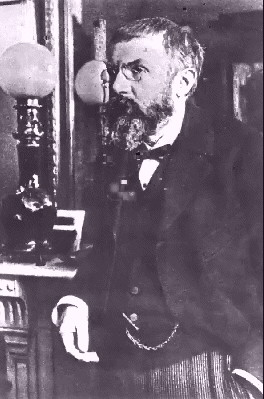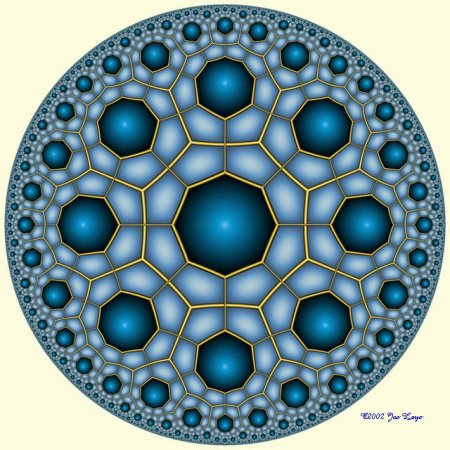A# Poincaré, (Jules) Henri (1854–1912)Fig 1. Henri Poincaré.Fig 2. Computer-generated tiling of a Poincaré disk by Jos Leys.

Henri Poincaré was a French mathematician and theoretical physicist who revolutionized celestial mechanics, inaugurating a rigorous treatment and initiating studies of the stability of the solar system. One of the greatest mathematicians of all time, Poincaré made significant advances in function theory, algebraic functions, algebraic geometry, algebra, number theory, algebraic topology, and above all, differential equations. He determined the shape of a rotating fluid subject only to gravity. He also wrote extensively on the philosophy of science, worked in mathematical physics, and developed many of the ideas and equations of the special theory of relativity independently of Albert Einstein and Hendrik Lorentz.

Poincaré, who taught for most of his career at the University of Paris (1881 to 1912), was sometimes referred to as "the last universalist," because of his huge published output on a wide variety of mathematics and mathematical physics. Strangely, he was also clumsy, absent-minded, and inept at simple arithmetic.

In order to translate problems in topology into questions in algebra, he devised (or discovered) so-called homotopy groups – quantities that capture the essence of multi-dimensional spaces in algebraic terms, and have the power to reveal similarities between them. He is most famous in mathematics because of his hypothesis known as the Poincaré conjecture, which was the first conjecture ever made in topology. The Poincaré conjecture holds that if any loop in a given three-dimensional space can be shrunk to a point, the space is equivalent to a sphere. It remained an unsolved problem until Grigori Perelman proved a complete proof in 2003.

## Poincaré disk

The Poincaré disk is the region inside (but not including) a bounding circle, where straight lines are defined to be either diameters of the bounding circle, or arcs of circles that are perpendicular to the bounding circle. The Poincaré disk is a model of hyperbolic geometry. The great flexibility it allows in specifying the angles of a triangle leads to an infinite variety of ways in which the disk can be tiled (Fig 2).